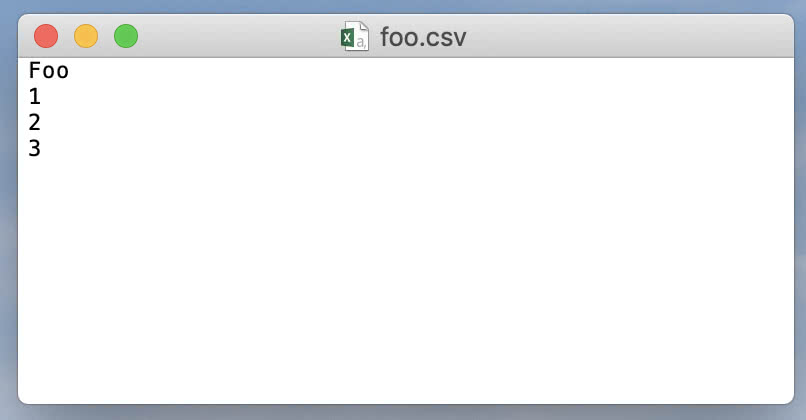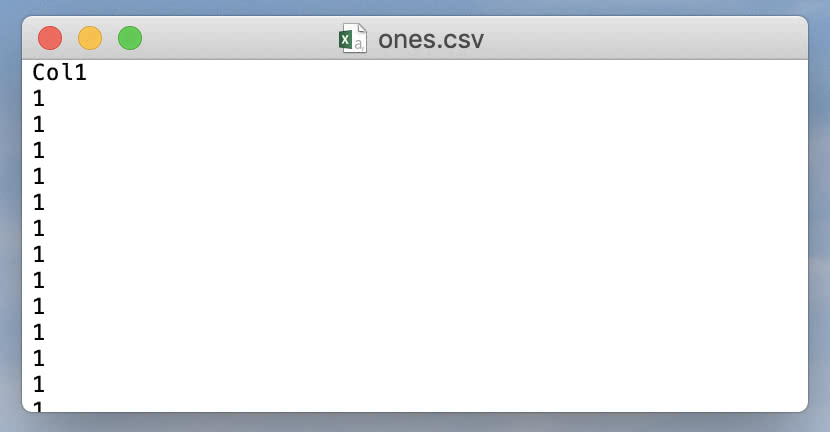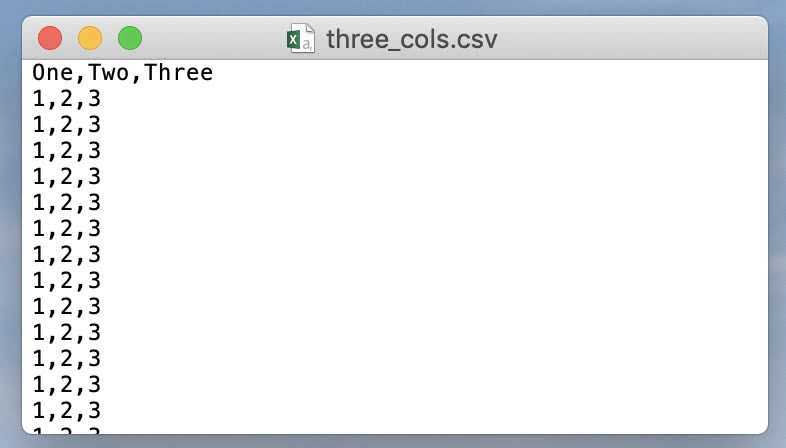# Reading And Writing CSV Files With C++

Contents

As a data scientist, reading and writing data from/to CSV is one of the most common tasks I do on the daily. R, my language of choice, makes this easy with `read.csv()` and `write.csv()` (although I tend to use `fread()` and `fwrite()` from the data.table package).

Hot Take. C++ is not R.

As far as I know, there is no CSV reader/writer built into the C++ STL. That’s not a knock against C++; it’s just a lower level language. If we want to read and write CSV files with C++, we’ll have to deal with File I/O, data types, and some low level logic on how to read, parse, and write data. For me, this is a necessary step in order to build and test more fun programs like machine learning models.

## Writing to CSV

We’ll start by creating a simple CSV file with one column of integer data. And we’ll give it the header Foo.

``````#include <fstream>

int main() {
// Create an output filestream object
std::ofstream myFile("foo.csv");

// Send data to the stream
myFile << "Foo\n";
myFile << "1\n";
myFile << "2\n";
myFile << "3\n";

// Close the file
myFile.close();

return 0;
}
``````Here, ofstream is an “output file stream”. Since it’s derived from ostream, we can treat it just like cout (which is also derived from ostream). The result of executing this program is that we get a file called foo.csv in the same directory as our executable. Let’s wrap this into a `write_csv()` function that’s a little more dynamic.

``````#include <string>
#include <fstream>
#include <vector>

void write_csv(std::string filename, std::string colname, std::vector<int> vals){
// Make a CSV file with one column of integer values
// filename - the name of the file
// colname - the name of the one and only column
// vals - an integer vector of values

// Create an output filestream object
std::ofstream myFile(filename);

// Send the column name to the stream
myFile << colname << "\n";

// Send data to the stream
for(int i = 0; i < vals.size(); ++i)
{
myFile << vals.at(i) << "\n";
}

// Close the file
myFile.close();
}

int main() {
// Make a vector of length 100 filled with 1s
std::vector<int> vec(100, 1);

// Write the vector to CSV
write_csv("ones.csv", "Col1", vec);

return 0;
}
``````Cool. Now we can use `write_csv()` to write a vector of integers to a CSV file with ease. Let’s expand on this to support multiple vectors of integers and corresponding column names.

``````#include <string>
#include <fstream>
#include <vector>
#include <utility> // std::pair

void write_csv(std::string filename, std::vector<std::pair<std::string, std::vector<int>>> dataset){
// Make a CSV file with one or more columns of integer values
// Each column of data is represented by the pair <column name, column data>
//   as std::pair<std::string, std::vector<int>>
// The dataset is represented as a vector of these columns
// Note that all columns should be the same size

// Create an output filestream object
std::ofstream myFile(filename);

// Send column names to the stream
for(int j = 0; j < dataset.size(); ++j)
{
myFile << dataset.at(j).first;
if(j != dataset.size() - 1) myFile << ","; // No comma at end of line
}
myFile << "\n";

// Send data to the stream
for(int i = 0; i < dataset.at(0).second.size(); ++i)
{
for(int j = 0; j < dataset.size(); ++j)
{
myFile << dataset.at(j).second.at(i);
if(j != dataset.size() - 1) myFile << ","; // No comma at end of line
}
myFile << "\n";
}

// Close the file
myFile.close();
}

int main() {
// Make three vectors, each of length 100 filled with 1s, 2s, and 3s
std::vector<int> vec1(100, 1);
std::vector<int> vec2(100, 2);
std::vector<int> vec3(100, 3);

// Wrap into a vector
std::vector<std::pair<std::string, std::vector<int>>> vals = {{"One", vec1}, {"Two", vec2}, {"Three", vec3}};

// Write the vector to CSV
write_csv("three_cols.csv", vals);

return 0;
}
``````Here we’ve represented each column of data as a `std::pair` of `<column name, column values>`, and the whole dataset as a `std::vector` of such columns. Now we can write a variable number of integer columns to a CSV file.

## Reading from CSV

Now that we’ve written some CSV files, let’s attempt to read them. For now let’s correctly assume that our file contains integer data plus one row of column names at the top.

``````#include <string>
#include <fstream>
#include <vector>
#include <utility> // std::pair
#include <stdexcept> // std::runtime_error
#include <sstream> // std::stringstream

std::vector<std::pair<std::string, std::vector<int>>> read_csv(std::string filename){
// Reads a CSV file into a vector of <string, vector<int>> pairs where
// each pair represents <column name, column values>

// Create a vector of <string, int vector> pairs to store the result
std::vector<std::pair<std::string, std::vector<int>>> result;

// Create an input filestream
std::ifstream myFile(filename);

// Make sure the file is open
if(!myFile.is_open()) throw std::runtime_error("Could not open file");

// Helper vars
std::string line, colname;
int val;

// Read the column names
if(myFile.good())
{
// Extract the first line in the file
std::getline(myFile, line);

// Create a stringstream from line
std::stringstream ss(line);

// Extract each column name
while(std::getline(ss, colname, ',')){

// Initialize and add <colname, int vector> pairs to result
result.push_back({colname, std::vector<int> {}});
}
}

// Read data, line by line
while(std::getline(myFile, line))
{
// Create a stringstream of the current line
std::stringstream ss(line);

// Keep track of the current column index
int colIdx = 0;

// Extract each integer
while(ss >> val){

// Add the current integer to the 'colIdx' column's values vector
result.at(colIdx).second.push_back(val);

// If the next token is a comma, ignore it and move on
if(ss.peek() == ',') ss.ignore();

// Increment the column index
colIdx++;
}
}

// Close file
myFile.close();

return result;
}

int main() {
// Read three_cols.csv and ones.csv
std::vector<std::pair<std::string, std::vector<int>>> three_cols = read_csv("three_cols.csv");
std::vector<std::pair<std::string, std::vector<int>>> ones = read_csv("ones.csv");

// Write to another file to check that this was successful
write_csv("three_cols_copy.csv", three_cols);
write_csv("ones_copy.csv", ones);

return 0;
}
``````

This program reads our previously created CSV files and writes each dataset to a new file, essentially creating copies of our original files.

## Going further

So far we’ve seen how to read and write datasets with integer values only. Extending this to read/write a dataset of only doubles or only strings should be fairly straight-forward. Reading a dataset with unknown, mixed data types is another beast and beyond the scope of this article, but see this code review for possible solutions.

Special thanks to papagaga and Incomputable for helping me with this topic via codereview.stackexchange.com.